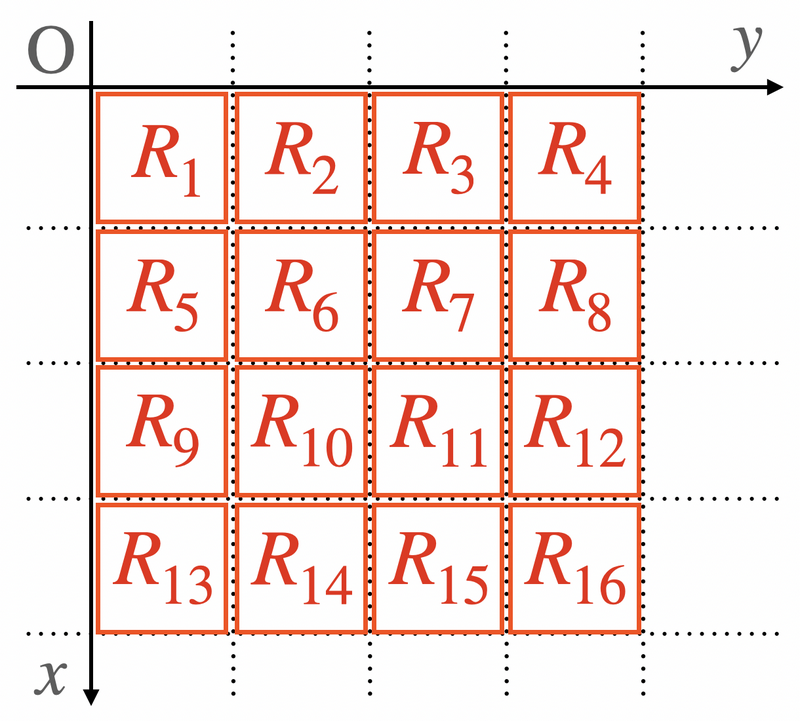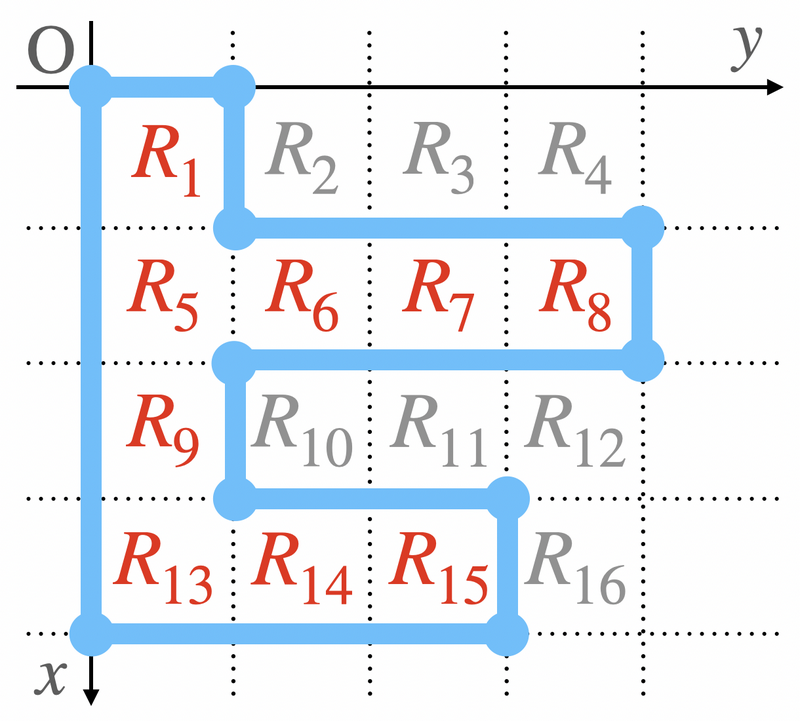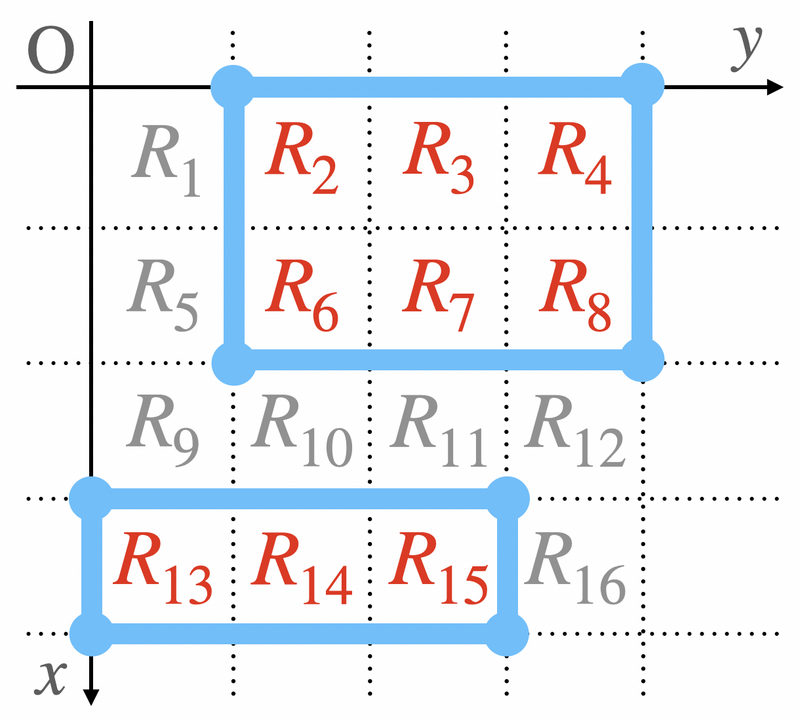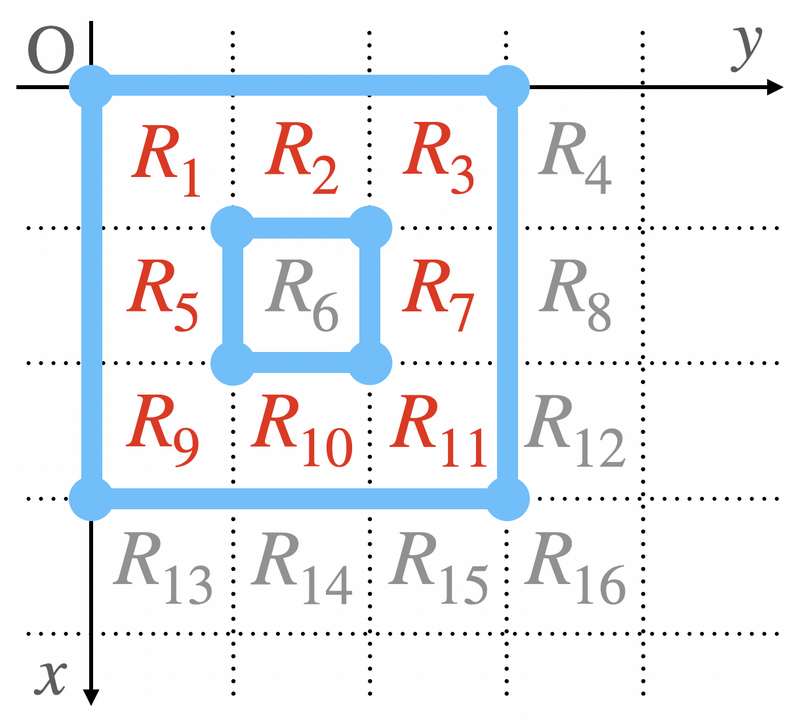Contest Duration: - (local time) (100 minutes) Back to Home

## E - Moat Editorial by en_translator

Consider the following $$16$$ regions $$R_1, R_2, \ldots,$$ and $$R_{16}$$.Once Takahashi determines a moat to build, each of $$16$$ regions belongs to either inside or outside the moat.
For example, in the following diagram, the $$16$$ regions are divided into two groups: those inside the moat, $$R_1, R_5, R_6, R_7, R_8, R_9, R_{13}, R_{14}, R_{15}$$, and those outside, $$R_2, R_3, R_4, R_{10}, R_{11}, R_{12}, R_{16}$$.Also, if two moats that Takahashi builds are different, then the divisions are also different.
Therefore, we can brute force through all the possible way to split $$16$$ regions into two groups, inside and outside, and check if “there exists a corresponding moat, and all the villages are included inside the moat.”

Note that divisions of regions like the following two diagrams does not correspond to a moat.posted:
last update: